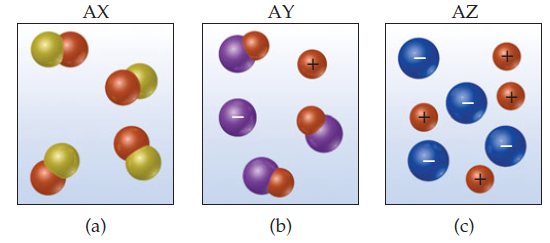×
Get Full Access to Chemistry: The Central Science - 12 Edition - Chapter 4 - Problem 2e
Get Full Access to Chemistry: The Central Science - 12 Edition - Chapter 4 - Problem 2e

×

# Visualizing ConceptsAqueous solutions of three differentISBN: 9780321696724 27

## Solution for problem 2E Chapter 4

Chemistry: The Central Science | 12th Edition

• Textbook Solutions
• 2901 Step-by-step solutions solved by professors and subject experts
• Get 24/7 help from StudySoup virtual teaching assistantsChemistry: The Central Science | 12th Edition

4 5 1 380 Reviews
11
3
Problem 2E

VISUALIZING CONCEPTS

Aqueous solutions of three different substances, AX, AY, and AZ, are represented by the three accompanying diagrams. Identify each substance as a strong electrolyte, weak electrolyte, or nonelectrolyte. [Section 4.1]Step-by-Step Solution:
Step 1 of 3

Biol/Chem5311 - Metabolism Pia Vogel office: 233, DLS lab: 221 phone: 214-768-1790 pvogel@smu.edu Thermodynamics Background Thermodynamics Background Free energy changes are additive Thermodynamics Background Now some chemistry Standard Free Energy Change and Equilibrium of a Reaction • Nucleophiles, electrophiles • Carbon-carbon, carbon-hydrogen bond cleavage, formation • Carbonyl chemistry • Isomerization, rearrangements, elimination • Group transfer reactions

Step 2 of 3

Step 3 of 3

## Discover and learn what students are asking

Calculus: Early Transcendental Functions : Evaluating Limits Analytically
?Finding a Limit In Exercises 5-36, find the limit. $$\lim _{x \rightarrow-3} x^{4}$$

Calculus: Early Transcendental Functions : Differentiation and Integration of Vector-Valued Functions
?Differentiation of Vector-Valued Functions In Exercises 1-6, find r’(t), $$\mathrm{r}\left(t_{0}\right)$$, and $$\mathrm{r’}\left(t_{0}\right)$$ for t

Statistics: Informed Decisions Using Data : Inference on the Least-Squares Regression Model and Multiple Regression
?Rents in New York The data in Problem 3 represent the square footage and rent for apartments in the borough of Queens and Nassau County, New York.

Unlock Textbook Solution